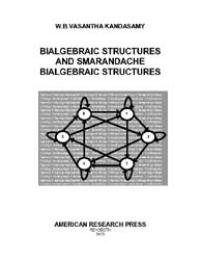•### Bialgebraic Structures and Smarandache Bialgebraic Structures

##### By: W. B. Vasantha Kandasamy

...belian or a commutative group. If the group G has only a finite number of elements we call G a group of finite order otherwise G is said to be of in... ...G is said to be of infinite order. If a b ≠ b a, for atleast a pair of elements a, b ∈ G, then we call G a non-commutative group. Notation: Let... ...up of G then o(H) is a divisor of o(G). Note: o(G) means the number of elements in G it will also be denoted by |G|. CAUCHY THEOREM (FOR ABELIA... ...ontain any non-trivial normal subloop. DEFINITION 1.1.4: If x and y are elements of a loop L, the commutator (x, y) is defined by xy = (yx) (x, y)... ... 〉 denotes the subloop generated by A. DEFINITION 1.1.5: If x, y, z are elements of a loop L an associator (x, y, z) is defined by (xy)z = (x(yz))... ..., fuzzy groups, and applications of fuzzy theory to the problems faced in chemical industries and cement industries. Currently, six Ph.D. scholars a...